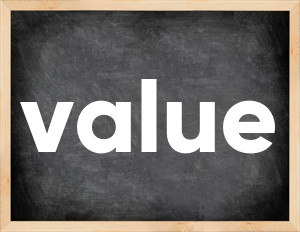# Value past tenseThe English verb 'value' is pronounced as [ˈvæljuː].
Related to: regular verbs.
3 forms of verb value: Infinitive (value), Past Simple - (valued), Past Participle - (valued).

## Here are the past tense forms of the verb value

👉 Forms of verb value in future and past simple and past participle.
❓ What is the past tense of value.

## Value: Past, Present, and Participle Forms

Base Form Past Simple Past Participle
value [ˈvæljuː]

valued [ˈvæljuːd]

valued [ˈvæljuːd]

## What are the 2nd and 3rd forms of the verb value?

🎓 What are the past simple, future simple, present perfect, past perfect, and future perfect forms of the base form (infinitive) 'value'?

### Learn the three forms of the English verb 'value'

• the first form (V1) is 'value' used in present simple and future simple tenses.
• the second form (V2) is 'valued' used in past simple tense.
• the third form (V3) is 'valued' used in present perfect and past perfect tenses.

## What are the past tense and past participle of value?

The past tense and past participle of value are: value in past simple is valued, and past participle is valued.

### What is the past tense of value?

The past tense of the verb "value" is "valued", and the past participle is "valued".

### Verb Tenses

Past simple — value in past simple valued (V2).
Future simple — value in future simple is value (will + V1).
Present Perfect — value in present perfect tense is valued (have/has + V3).
Past Perfect — value in past perfect tense is valued (had + V3).

### value regular or irregular verb?

👉 Is 'value' a regular or irregular verb? The verb 'value' is regular verb.

## Examples of Verb value in Sentences

•   Paul values the time he spends with her doughters. (Present Simple)
•   Shennon valued his privacy. (Past Simple)
•   The committee states that it has valued the property at original cost. (Present Perfect)
•   Do you know how much you are valued as an employee? (Present Simple)
•   He valued money above everything. (Past Simple)
•   I need to value her privacy. (Present Simple)
•   This paintings are highly valued by collectors. (Present Simple)
•   He was valued for his skill in finding solutions. (Past Simple)
•   We decided to get the sculpture valued. (Past Simple)
•   Does parenting is fuly valued by society? (Present Simple)

Along with value, words are popular defeat and control.

Verbs by letter: , , , , , , , , , , , , , , , , , , , , , , , , .